# Change Nickname

Current Nickname:

Certifications

Certificate Issued at Valid until Serial No. File
Serial No. Valid until

Not having one? Apply now! >>>

Invoice

ProductName CreateTime ID Price File
ProductName CreateTime ID Price File

# Skip-gram

## Background

Skip-gram (SG) model, or continuous Skip-gram model, is originated from the Word2Vec algorithm in NLP (Natural Language Processing) field. Word2Vec embeds words to vector space, and words that are semantically similar have closer locations in vector space. Word2Vec was proposed by T. Mikolov et al. of Google in 2013, it actually includes two models - Skip-gram and CBOW. Related materials of the algorithm are:

In the graph embedding field, DeepWalk, which came out in 2014, was the first one to apply Skip-gram model to the training of vector representations of nodes in graph. The key idea is: node is viewed as 'word', node sequence generated by random walk is viewed as 'sentence', a series of node sequences forms the 'corpus'; input the 'corpus' into Skip-gram model for training to get the vector representations of nodes. Related material of the algorithm is:

Graph embedding algorithms that were proposed later, such as Node2Vec and Struc2Vec, have improved DeepWalk while still using Skip-gram.

We will introduce Skip-gram using the natural language of its original application as an example.

## Model Overview

The basic mechanism of Skip-gram is to predict several context words of a given target word. As shown in the diagram below: input word w(t) into model, then it outputs 4 context words of w(t): w(t-2), w(t-1), w(t+1), and w(t+2); where +/- represents context before and after the target word, the number of output context words is adjustable.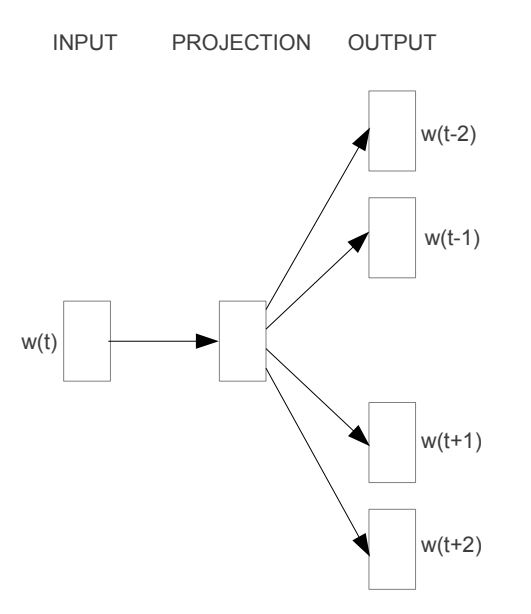It is noteworthy that the real goal of Skip-gram is not to conduct the prediction, but to obtain the weighted matrix contained in the mapping relation (PROJECTION in above diagram), the weighted matrix represents the vector representations of words.

## Model Training — Original Form

Training of Skip-gram model adopts the backpropagation (BP) algorithm, please refer to the documentation of backpropagation if you are not familiar with it.

### Corpus

Assume that the corpus contains 10 words:

graph, is, a, good, way, to, visualize, data, very, at

and a series of sentences of these words, one of the sentences is:

Graph is a good way to visualize data.

### Sliding Window Sampling

Skip-gram model uses a sliding window to produce samples: a window sequentially takes each word in the sentence as target and combine the target word with every word before and after the target word within `window_size` steps.

Shown below are the sampling results when `window_size` = 1. Please note the distance between the context word and target word within the window plays no role when `window_size` > 1.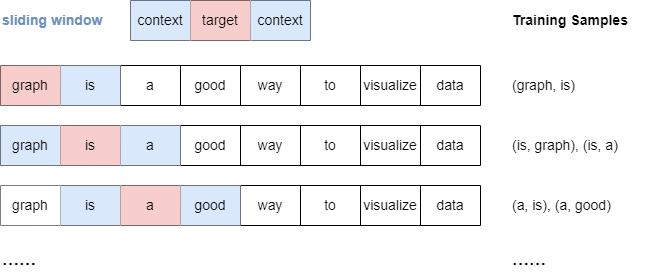### One-hot Encoding

Words cannot enter into the model directly, they have to be complied into machine codes. Skip-gram adopts one-hot encoding to preprocess words. In our example, the one-hot encodings of the 10 words are: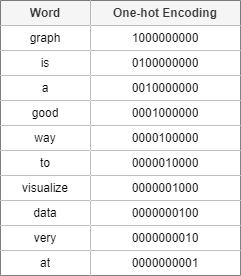### Model Description

Below is a detailed illustration of Skip-gram model: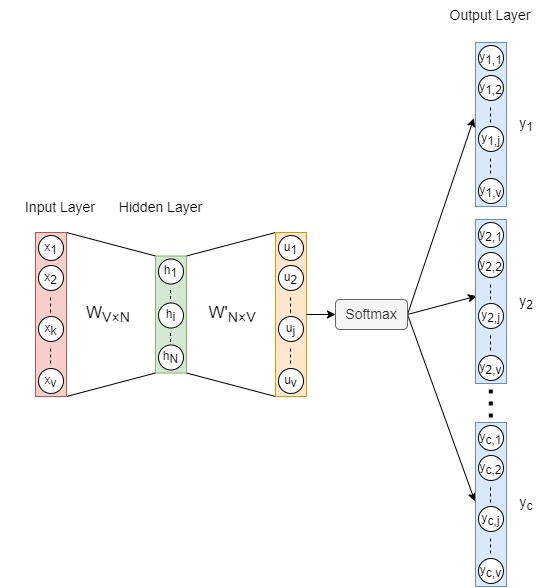where,

• V：number of words in corpus
• N：dimension of the hidden layer vectors, i.e., dimension of the embedding vector of words
• C：number of output vectors, i.e., number of context words, which is related to the size of sampling window
• x：1×V one-hot vector of the input word
• h：1×N vector in the hidden layer
• u：output vector before being processed by Softmax function
• y: actual output vector after being processed by Softmax; C same vectors y (denoted as yc) are output, each yc represents a context word
• W: V×N weighted matrix between input layer and hidden layer
• W': N×V weighted matrix with between hidden layer and output layer

About weighted matrix: Each row of matrix W (vw) is the N-dimensional embedding vector of a word (the order of rows is consistent with the order of one-hot encodings), vw is called the input vector of word. Each column of matrix W' (v'w) can be regarded as another N-dimensional vector representation of word (the order of columns is consistent with the order of one-hot encodings), v'w is called the output vector of word. Both matrices are initialized with random values. W and W' are different, W' is not the transpose of W. The training of model is to update weights of both matrices recursively with the ultimate goal of obtaining matrix W.

Indeed, the design of two independent matrices indicates that each word would have two representations: input vector vw is regarded as the representation when taking the word as a target, and output vector v'w is the representations when the word is being as context. The fact is that this separation makes the computation easier and more accurate.

About Softmax function：Softmax is an activation function, which normalizes a numeric vector to a probability distribution vector with the sum of all probabilities as 1. The formula is: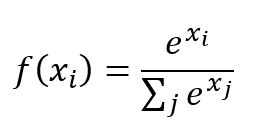### Forward Propagation

Take the training samples (is, graph) and (is, a) as example: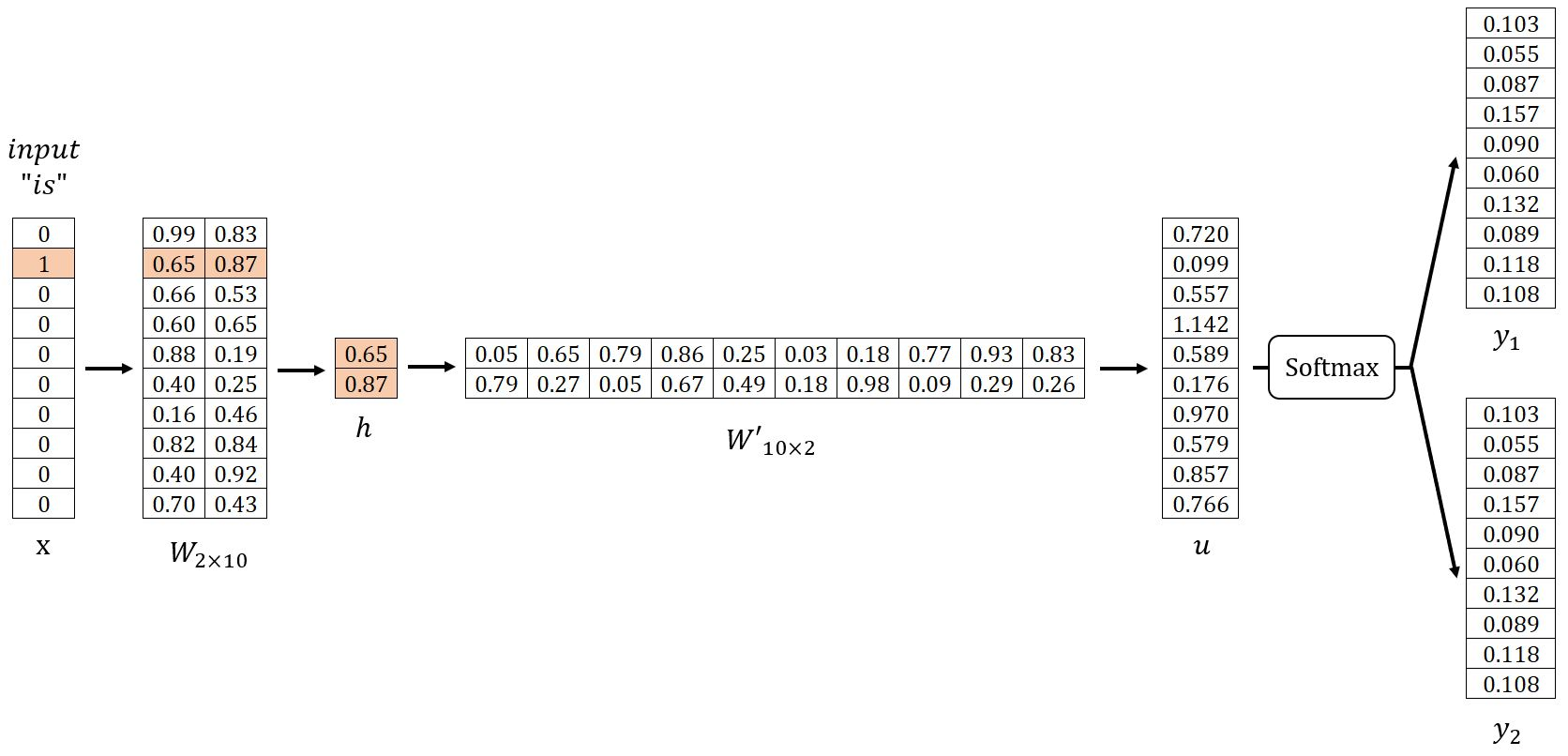#### Input Layer → Hidden Layer

Hidden layer vector h is obtained by multiplying vector x and matrix W's transpose WT. Since x is a one-hot vector, i.e., xk = 1 while all other components are 0, this operation can be regarded as to extract the vector in the k-th row from matrix W without calculation.

#### Hidden Layer → Output Layer

Vector u is obtained by multiplying vector h and the transpose of W':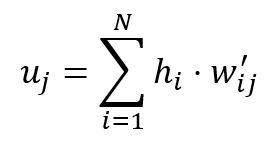Every component (uj) of vector u can be taken as a score, and it produces the final output vector y with Softmax: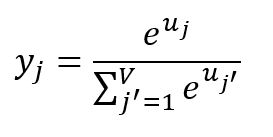Each component of vector y represents the probability of each word being output when the input x is given, the sum of all probabilities is 1 (same order with one-hot encodings).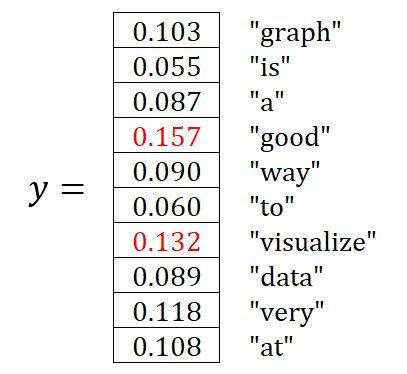In this example, the two words with the highest probabilities are 'good' and 'visualize', which are the two context words the model outputs this time. They are different from the expected outputs 'graph' and 'a', and we will then adjust weights of matrices W and W' via backpropagation.

### Loss Function

#### One-word Context

We start from the simplest version, we assume that model only outputs one vector y. yj represents the j-th component of vector y, i.e., the probability of outputting the j-th word in the corpus. Suppose that the expected output is the j*-th word, then the goal of model training would be to maximize yj*:In machine learning, it is often easier to target at minimization rather than maximization, hence we perform some transform to the goal above: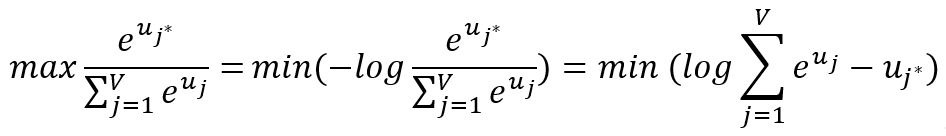The goal is not affected by taking the logarithm of yj, and since yj ∈(0,1), its logarithm is negative, thus we can transform it into a minimization problem.

Then we get the loss function of the model: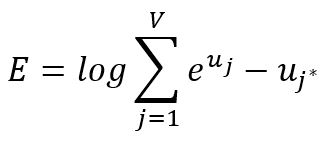Take the partial derivative of E with regard to uj, and define the result as ej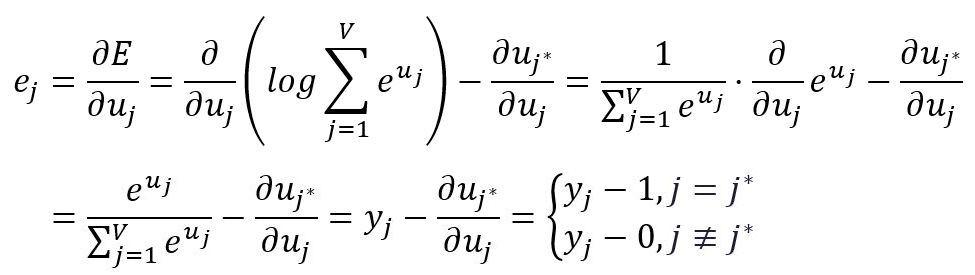It is advised that readers be familiar with calculating derivatives of logarithmic and exponential functions.

Consider about the real meaning of ej. Take the training sample (is, graph) as example, the expected output 'graph' is at the first position, hence we have: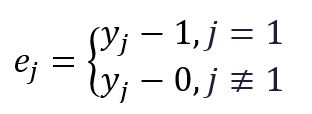The output vector y in this example is as followed, where y4 is the greatest, i.e., the model actually outputs the 4th word 'good'. And we notice that the calculation of ej is equivalent to deduct the one-hot vector from the output vector y, therefore, ej is the prediction error of the model.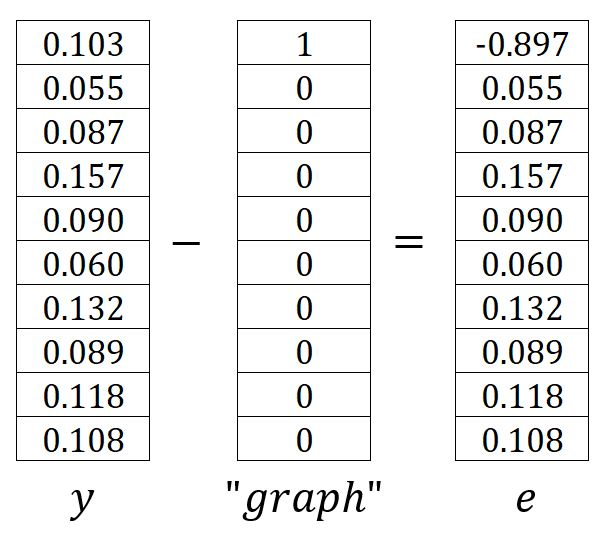#### Multi-word Context

When outputing multiple vectors, yc,j represents the j-th component of the c-th vector yc, similarly, it is the probability of thr j-th word in the corpus being output. As aforementioned, all sampling results in the sliding window are equal. For C context words, the goal of model training is to maximize every probability of the context word, i.e., to maximize the product of these probabilities: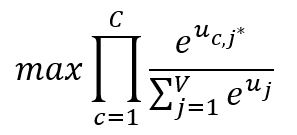Similarly, we get the loss function E after the transformation: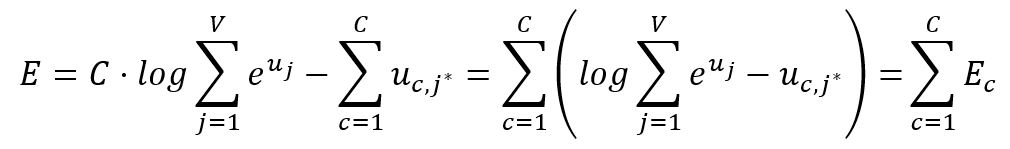Take the partial derivative of E with regard to uj: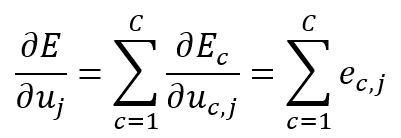This means that when outputting multiple context words, the prediction error of the model is equal to the sum of errors of predicting single context word.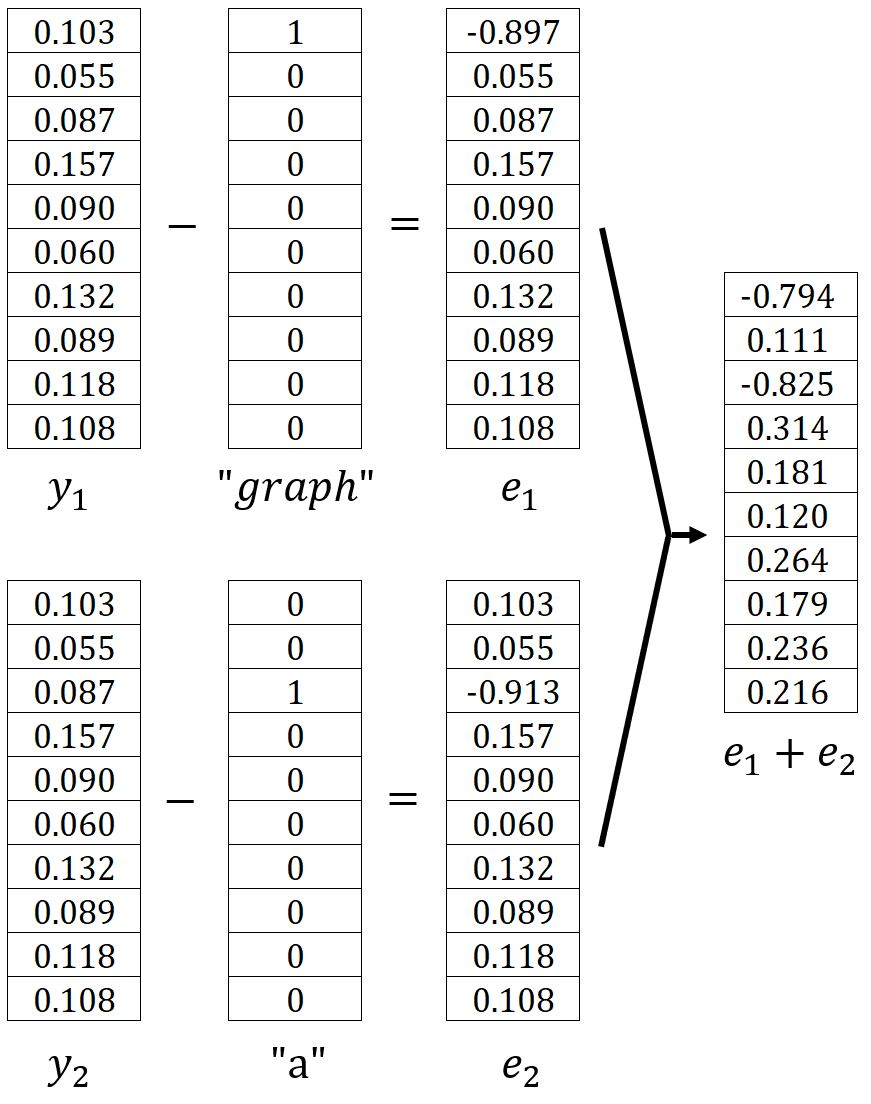### Backpropagation

During backpropagation, we adjust parameters of the model, i.e., weights of matrices W and W'. Skip-gram adopts stochastic gradient descent (SGD) to update weights. Samples above are still used in this section.

#### Output Layer → Hidden Layer

Matrix W' is between the output layer and hidden layer, we need to calculate how much influence w'ij imposes on E by taking the partial derivative of E with regard to w'ij by the chain rule: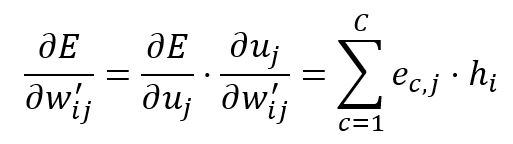Therefore, according to learning rate η∈(0,1), we adjust w'ij: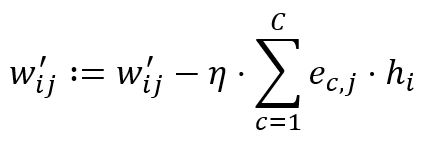Suppose η = 0.4, for instance, weight w'14 = 0.86 and w'24 = 0.67 are updated to:

w'14 := w'14 - η*(e1,4+e2,4)*h1 = 0.86 - 0.4*0.314*0.65 = 0.78
w'24 := w'24 - η*(e1,4+e2,4)*h2 = 0.67 - 0.4*0.314*0.87 = 0.56

All weights of matrix W' will be updated, which means that all the output vectors of words will be updated.

#### Hidden Layer → Input Layer

Vector h can be simply obtained by looking up the table, but in order to calculate the partial derivative, we can use the equivalent formula below: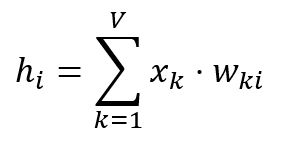Matrix W is between the hidden layer and input layer, we can calculate how much influence wki imposes on E by taking the partial derivatie of E with regard to wki by the chain rule: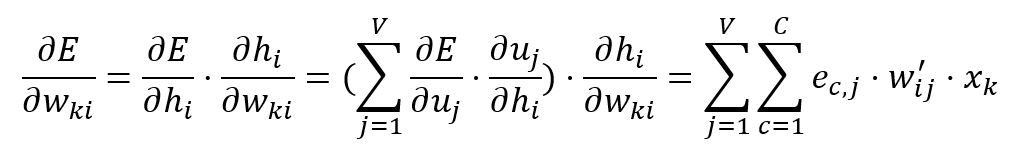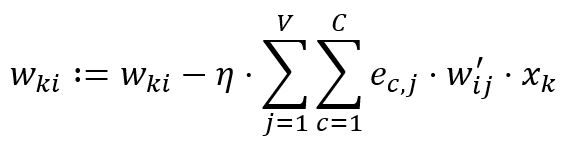Vector x is a one-hot vector, only one component xk = 1 and others are all 0, hence only weights in k-th row of matrix W, wki, will be updated and others stay unchanged. In this example, only w21 = 0.65 and w22 = 0.87 are updated：

first,

∂E/∂w21 = (e1,1+e2,1)*w'11*x2 + (e1,2+e2,2)*w'12*x2 + ... + (e1,10+e2,10)*w'1,10*x2 = -0.794*0.05*1 + 0.111*0.65*1 + ... + 0.216*0.83*1 = 0.283
∂E/∂w22 = (e1,1+e2,1)*w'21*x2 + (e1,2+e2,2)*w'22*x2 + ... + (e2,10+e2,10)*w'1,10*x2 = -0.794*0.79*1 + 0.111*0.27*1 + ... + 0.216*0.26*1 = 0.081

then,

w21 := w21 - η*∂E/∂w21 = 0.65 - 0.4*0.283 = 0.54
w22 := w22 - η*∂E/∂w22 = 0.87 - 0.4*0.081 = 0.84

Only one row of matrix W will be updated, i.e., only the input vector of the target word will be updated.

## Optimize Computation Efficiency

About optimizing the training process for Skip-gram model and making the computation complexity feasible in practice, please see Skip-gram Model Optimization.